## Example Questions

### Example Question #13 : Triangles

The three angles in a triangle measure 3x, 4x + 10, and 8x + 20. What is x?

25

30

20

15

10

10

Explanation:

We know the angles in a triangle must add up to 180, so we can solve for x

3x + 4x + 10 + 8x + 20 = 180

15x + 30 = 180

15x = 150

x = 10

### Example Question #14 : Triangles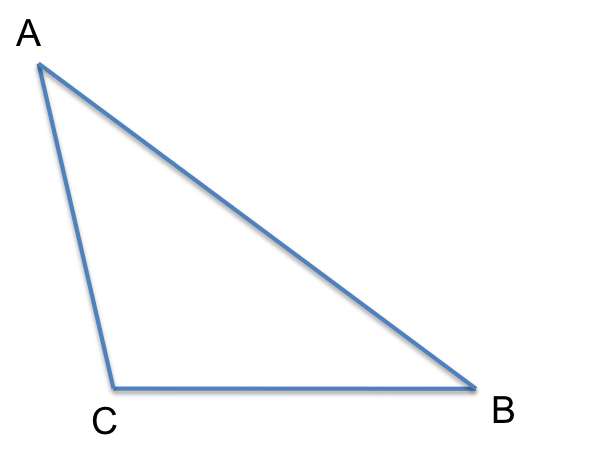In triangle ABC, AB=6, AC=3, and BC=4.

Quantity A                               Quantity B

angle C                   the sum of angle A and angle B

Quantity A is greater

Quantity A and B are equal

The relationship cannot be determined from the information given.

Quantity B is greater

Quantity A is greater

Explanation:

The given triangle is obtuse. Thus, angle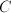is greater than 90 degrees. A triangle has a sum of 180 degrees, so angle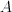+ angle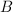+ angle= 180. Since angle C is greater than 90 then angle+ anglemust be less than 90 and it follows that Quantity A is greater.

### Example Question #15 : Triangles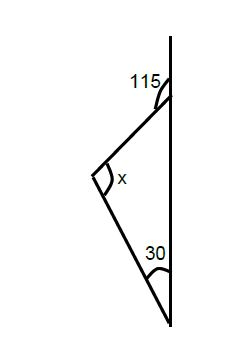In the figure above, what is the value of angle x?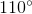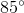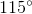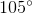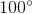Explanation:

To find the top inner angle, recognize that a straight line contains 180o; thus we can subtract 180 – 115 = 65o. Since we are given the other interior angle of 30 degrees, we can add the two we know: 65 + 30 = 95o.

180 - 95 = 85

Tired of practice problems?

Try live online GRE Math prep today.